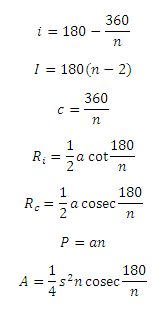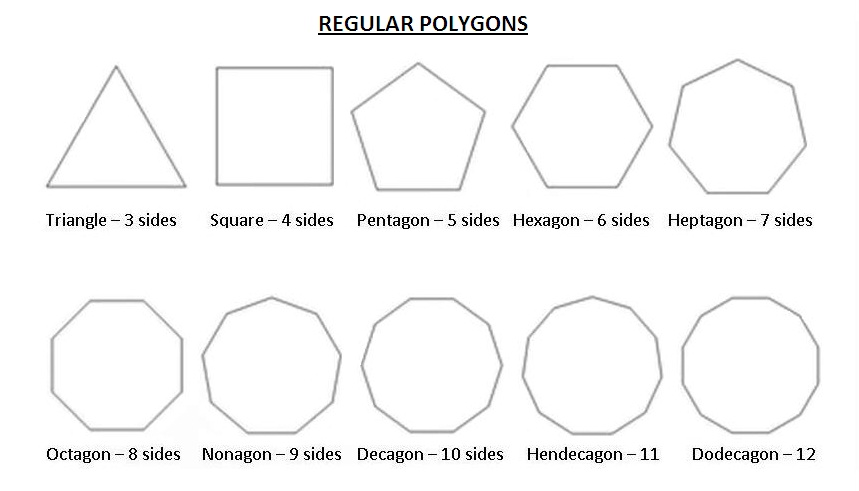# Regular Polygon Calculator

This CalcTown calculator calculates the various parameters of a regular polygon.

#### Result

degrees
degrees
degrees
m
m
m
m2Where,

a = length of each side of the polygon

n = no. of sides of the regular polygon

c = central angle

i = interior angle

I = sum of interior angles

Ri = in-radius of the regular polygon

Rc = circumradius of the regular polygon

P = perimeter of the regular polygon

A = area of the regular polygon